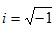Division

In order to divide complex numbers, write the problem in fractional form. Because, when dividing complex numbers there is a radical sign in the denominator of the fraction and it is therefore necessary to rationalize the denominator.

The purpose of rationalizing the denominator is to eliminate the radical sign in the denominator. In complex numbers this is achieved by using conjugates. The conjugate of a complex number a + bi is the complex number a - bi. For example, the conjugate of 4 + 2i is 4 - 2i. Notice that only the sign of the bi term is changed. The conjugate of 4i + 3 is not 4i - 3, but -4i + 3 because the sign on the bi term must change, not the a term.